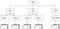Keras: Deep learning for humans.

Solutions to common problems faced when using Keras generators.

Keras is a great high-level library which allows anyone to create powerful machine learning models in minutes.

Note: This post assumes that you have at least some experience in using Keras.

Keras has this ImageDataGenerator class which allows the users to perform image augmentation on the fly in a very easy way. You can read about that in Keras’s official documentation.

The ImageDataGenerator class has three methods flow(), flow_from_directory() and flow_from_dataframe() to read the images from a big numpy array and folders containing images.

We will discuss only about flow_from_directory() in this blog post.

Download the train dataset and test dataset, extract them into 2 different folders named as “train” and “test”. The train folder should contain ‘n’ folders each containing images of respective classes. For example, In the Dog vs Cats data set, the train folder should have 2 folders, namely “Dog” and “Cats” containing respective images inside them.

Create a validation set, often you have to manually create a validation data by sampling images from the train folder (you can either sample randomly or in the order your problem needs the data to be fed) and moving them to a new folder named “valid”. If the validation set is already provided, you could use them instead of creating them manually.The directory structure for a binary classification problem

As you can see in the above picture, the test folder also should contain a single folder inside which all the test images are present(Think of it , this is there because the flow_from_directory() expects at least one directory under the given directory path.

The folder names for the classes are important, name(or rename) them with respective label names so that it would be easy for you later.

Once you set up the images into the above structure, you are ready to code!

Here are the most used attributes along with the flow_from_directory() method.

`train_generator = train_datagen.flow_from_directory(    directory=r"./train/",    target_size=(224, 224),    color_mode="rgb",    batch_size=32,    class_mode="categorical",    shuffle=True,    seed=42)`
• The directory must be set to the path where your ‘n’ classes of folders are present.
• The target_size is the size of your input images, every image will be resized to this size.
• color_mode: if the image is either black and white or grayscale set “grayscale” or if the image has three colour channels, set “rgb”.
• batch_size: No. of images to be yielded from the generator per batch.
• class_mode: Set “binary” if you have only two classes to predict, if not set to“categorical”, in case if you’re developing an Autoencoder system, both input and the output would probably be the same image, for this case set to “input”.
• shuffle: Set True if you want to shuffle the order of the image that is being yielded, else set False.
• seed: Random seed for applying random image augmentation and shuffling the order of the image.
`valid_generator = valid_datagen.flow_from_directory(    directory=r"./valid/",    target_size=(224, 224),    color_mode="rgb",    batch_size=32,    class_mode="categorical",    shuffle=True,    seed=42)`
• Same as train generator settings except for obvious changes like directory path.
`test_generator = test_datagen.flow_from_directory(    directory=r"./test/",    target_size=(224, 224),    color_mode="rgb",    batch_size=1,    class_mode=None,    shuffle=False,    seed=42)`
• directory: path where there exists a folder, under which all the test images are present. For example, in this case, the images are found in /test/test_images/
• batch_size: Set this to some number that divides your total number of images in your test set exactly.
Why this only for test_generator?
Actually, you should set the “batch_size” in both train and valid generators to some number that divides your total number of images in your train set and valid respectively, but this doesn’t matter before because even if batch_size doesn’t match the number of samples in the train or valid sets and some images gets missed out every time we yield the images from generator, but it would be sampled the very next epoch you train.
But for the test set, you should sample the images exactly once, no less or no more. If Confusing, just set it to 1(but maybe a little bit slower).
• class_mode: Set this to None, to return only the images.
• shuffle: Set this to False, because you need to yield the images in “order”, to predict the outputs and match them with their unique ids or filenames.

# Let’s Train, evaluate and predict!

## Fitting/Training the model

`STEP_SIZE_TRAIN=train_generator.n//train_generator.batch_sizeSTEP_SIZE_VALID=valid_generator.n//valid_generator.batch_sizemodel.fit_generator(generator=train_generator,                    steps_per_epoch=STEP_SIZE_TRAIN,                    validation_data=valid_generator,                    validation_steps=STEP_SIZE_VALID,                    epochs=10)`

## Evaluate the model

`model.evaluate_generator(generator=valid_generator,steps=STEP_SIZE_VALID)`

Since we are evaluating the model, we should treat the validation set as if it was the test set. So we should sample the images in the validation set exactly once(if you are planning to evaluate, you need to change the batch size of the valid generator to 1 or something that exactly divides the total num of samples in validation set), but the order doesn’t matter so let “shuffle” be True as it was earlier.

## Predict the output

`STEP_SIZE_TEST=test_generator.n//test_generator.batch_sizetest_generator.reset()pred=model.predict_generator(test_generator,steps=STEP_SIZE_TEST,verbose=1)`
• You need to reset the test_generator before whenever you call the predict_generator. This is important, if you forget to reset the test_generator you will get outputs in a weird order.
`predicted_class_indices=np.argmax(pred,axis=1)`

now predicted_class_indices has the predicted labels, but you can’t simply tell what the predictions are, because all you can see is numbers like 0,1,4,1,0,6…
and most importantly you need to map the predicted labels with their unique ids such as filenames to find out what you predicted for which image.

`labels = (train_generator.class_indices)labels = dict((v,k) for k,v in labels.items())predictions = [labels[k] for k in predicted_class_indices]`

Finally, save the results to a CSV file.

`filenames=test_generator.filenamesresults=pd.DataFrame({"Filename":filenames,                      "Predictions":predictions})results.to_csv("results.csv",index=False)`

Written by

## Vijayabhaskar J

#### Machine Learning Enthusiast

Welcome to a place where words matter. On Medium, smart voices and original ideas take center stage - with no ads in sight. Watch
Follow all the topics you care about, and we’ll deliver the best stories for you to your homepage and inbox. Explore
Get unlimited access to the best stories on Medium — and support writers while you’re at it. Just \$5/month. Upgrade